|

# 继续突破,格莱美追梦2019!

第二期销售精英特训营暨315全国联动启动大会圆满结束！

2019年虽已过去3个月，但对陶瓷行业来说才只是开端，2019年的陶瓷市场充满机遇，也充满挑战与考验，那么在2019年我们如何熟练运用营销技巧化机遇成为业绩增长的助力呢？

这次精英特训营带给你全新的陶瓷营销知识，颠覆你的传统观念，教你玩转陶瓷行业社群营销技巧，让你在2019年大获全胜！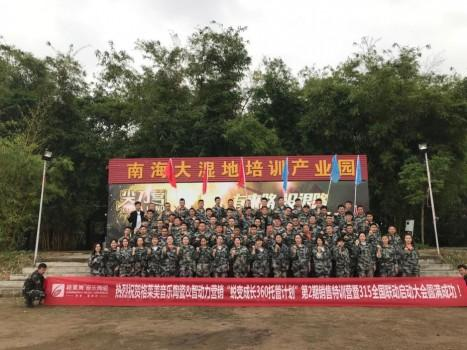格莱美音乐陶瓷“蜕变成长360托管计划”第2期销售精英特训营暨315全国联动启动大会于3月5号—7号燃情开启!

意气风发，荣誉归来

格莱美音乐陶瓷2018年业绩超额逆增长离不开格莱美全体经销商的贡献，今天，格莱美家人们带着荣誉、带着2019年的新目标归来了！

来自全国各地的格莱美家人们陆陆续续回到格莱美总部展厅，有序地签到，并已作好加入格莱美第二期销售精英特训营的准备。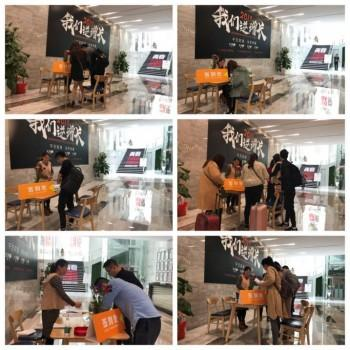▲欢迎格莱美家人们回家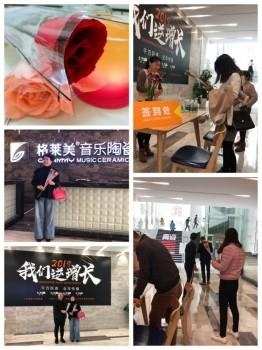诱人的玫瑰花、可口的下午茶、真诚的问候祝福、贴心的服务.....暖化了格莱美家人们的心，回家是一件多么美好的事啊！

路途虽远，格莱美家人们却依旧精神抖擞，毫无疲惫感。依旧用最专业的知识、最严谨的态度挑选格莱美2019年新产品，以产品为导向，为2019年格莱美音乐陶瓷进一步开拓市场提前作准备。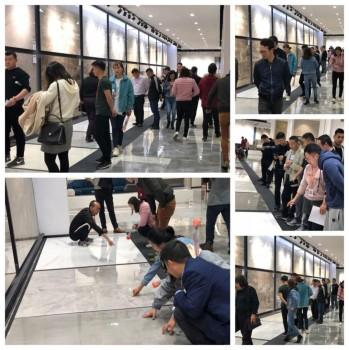夕阳落下，欢快的时光悄然过去，格莱美家人们收拾行囊，再次启程，前往南海大湿地公园训练基地，参加接下来的全封闭销售精英特训营的拓展培训。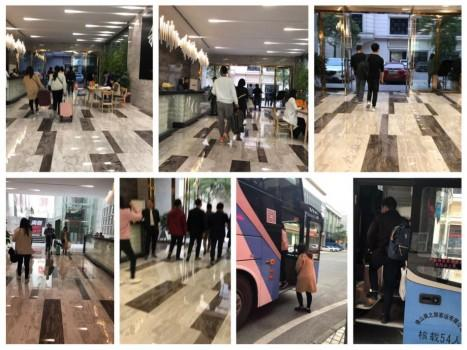整齐划一，严阵以待

虽初到南海大湿地公园训练基地，格莱美的家人们很快适应了陌生的环境，迅速换上戎装，集结队伍，以较佳的状态迎接后面未知的挑战！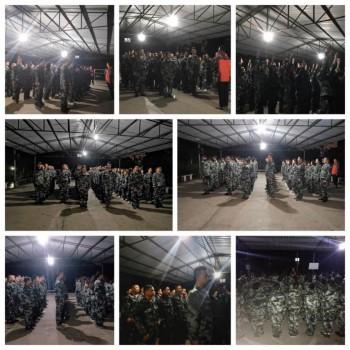站姿挺拔、队伍整齐划一，无不透露出格莱美家人们对本期销售精英特训营势必全力以赴的决心，也让我们看到了格莱美团队是一个优秀、素质良好的精英团队。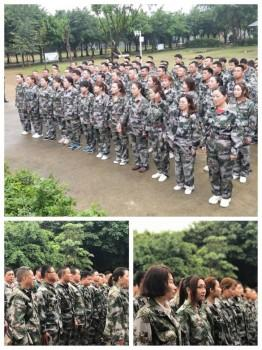团队行动，以质取胜

大家虽来自天南地北，可都是格莱美的家人，一直为打造格莱美音乐陶瓷成为陶瓷行业较好的品牌的共同目标而努力，自有一股亲切感，经过一系列的破冰活动，格莱美的家人们逐渐相互熟悉起来，成为相互信任、并肩作战的队友。

一个个团队户外拓展活动打破传统的培训模式，让格莱美的家人们走出仅听、看、学，直接参与到整个过程中去。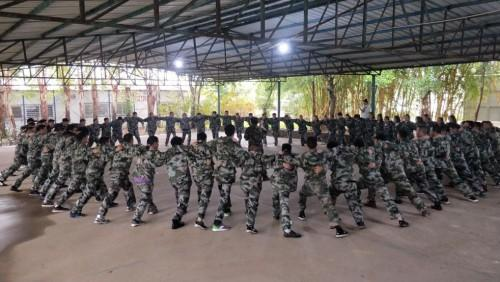在看似简单的团建活动中，格莱美家人们展现出良好的团队意识，懂得相互配合、相互信任、相互支持，快速、高效完成一个个任务。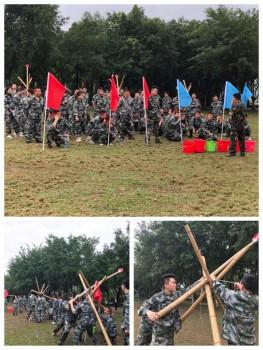在陶瓷行业亦如此，良好的销售团队是业绩保障的重要基础，格莱美一直致力于打造高效、一流的团队，为广大客户带去较好的产品、提供较好的服务！

即学即用，技能满级

富有挑战性、趣味性的户外拓展活动结束后，格莱美的家人们就立即转战课堂，如此紧凑的流程安排，更加激发格莱美家人们的求知欲、征服感！

课堂上，行业内著名营销专家韩军博士，以独特的授课方式、颠覆传统课堂培训模式，用生动形象的例子，传授了从微信爆破升级到社群深度运营，如何全新升级7大社群决战系统打造强IP体系，以此抵抗市场疲惫，实现弯道超车的一系列优质干货！

格莱美的家人怎会错过如此有价值的内容？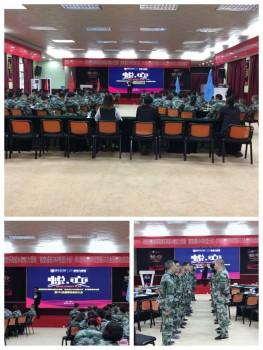学以致用才能较大发挥课堂培训的价值，课后，格莱美家人们面对充满挑战又十分具有检验性的魔王通关，毫不畏惧，化理论知识为实战技巧，熟练运用，用实力说话，优质作答，顺利通关！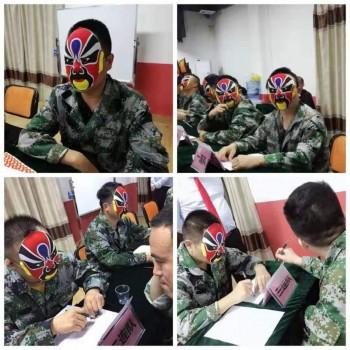在上半场的课堂培训和魔王通关中，格莱美家人们展现出强大的学习能力和优质诠释出学以致用的内涵！

格莱美家人们稍作调整，立即“满血复活”全身心投入到下半场课堂培训中去，因为学无止境是所有格莱美家人们的一直追求！

社群营销转换系统、社群圈养系统、社群营销裂变系统...... 这一系列的陌生名词让格莱美的家人们兴奋起来，聚精会神地听着，不错过一丝一毫的专业营销知识！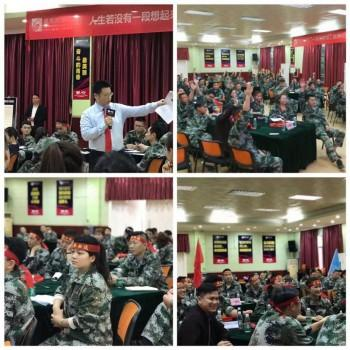跟着总战略走，继续强势突破

优秀经销商分享将课堂培训推向高潮，重庆优秀经销商王磊在分享的《一天30万是如何做到的》中表示，要选择较好的店面位置、用最精的店面展示和较好的产品打开当地的市场，主销售渠道一定要是当地No.1渠道，这样才会把销售额做上去！

石家庄优秀经销商王磊分享了《如何在活动期间突破160%销售额》，他提到超额完成销售额离不开格莱美总部战略的支撑，跟着总部思路走，在销售中有“底气”，给顾客营造踏实的购买氛围，采取一系列优惠措施，突破销售额不再是空谈！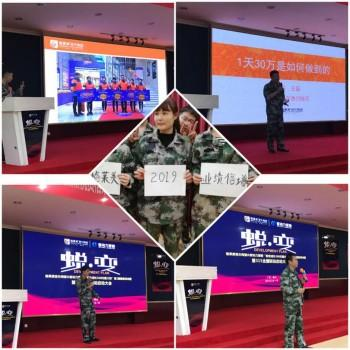部分优秀经销商也纷纷上台分享了自己在销售格莱美瓷砖上取得的佳绩，向大家传授了一些经验与技巧！

如此漂亮的业绩，让我们看到格莱美音乐陶瓷极具生命力，备受广大客户的喜爱，跟着总部战略走，实现新的突破！

315买砖不用钱，引爆全国

说干就干，由总部操盘的“315买砖不用钱”全国联动启动大会正式开启，“买砖不用钱”打破传统消费观念，充分引起消费者的关注，激发消费者的购买欲，再配以一套套可行的方案和一系列优惠措施，势必引爆全国。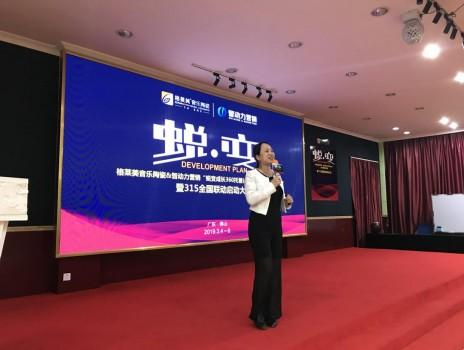格莱美的家人们也通过喝酒宣誓、军令状签约、PK战书等表明决心。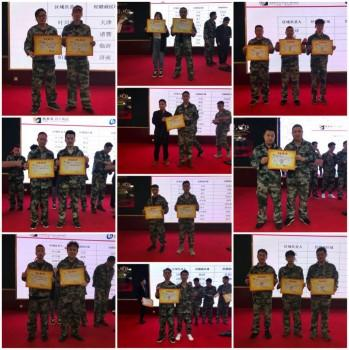相信，经过系统的培训、学习，对课程的熟练掌握和高效的作战能力，格莱美的家人们对接下来的“315买砖不花钱”全国联动大会必定充满信心，一冲到底，大获佳绩！

轻松一刻，满“载”而归

紧张、一气呵成课程培训结束了，格莱美的家人们终于可以轻松一刻了！

虽天气不佳，可格莱美的家人们依旧热情高涨，用欢声笑语燃爆全场！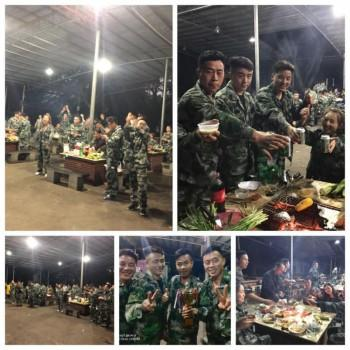各小组精心准备的节目，让大家欢笑不止，格莱美的家人们是如此多才多艺、能歌善舞！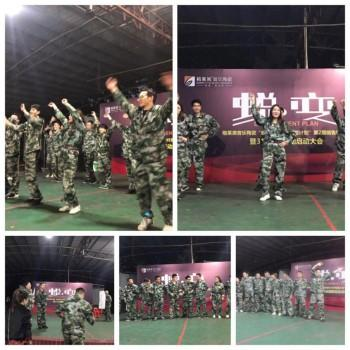接着，格莱美音乐陶瓷董事长康东先生分享多次的创业经历引起大家的共鸣。康东先生细细讲述了格莱美音乐陶瓷就像他的孩子那般，看着她成长与蜕变，成为陶瓷行业的一匹黑马，这过程中经历了许多艰难与险阻，始终不言弃，最终收获了许多时刻成功的喜悦与甜蜜。

康东先生表示，现格莱美音乐陶瓷已稳占一定的陶瓷市场份额；但仍需继续向前走，未来格莱美音乐陶瓷会继续脚踏实地、一步一脚印，成为陶瓷行业最终生存者！

最后，康东先生共勉大家：我们要认清自己的定位，在市场上精耕细作，做好每一个店的零售渠道；企业要营造简单、纯粹、快乐、高效、可信赖的文化氛围！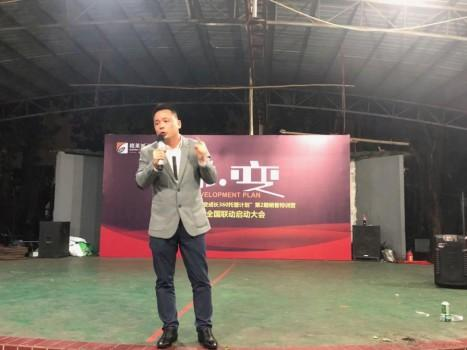▲格莱美音乐陶瓷 董事长 康东先生

同时，格莱美的家人们也分享了自己对这几天特训的感想，有的收获了新友情，有的收获了知识，有的收获了坚持......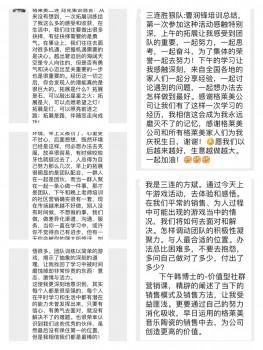荣誉时刻来了，排名前列期全国联动优秀经销商纷纷上台领奖，展示出全国联动活动的效果显著。让全国联动活动成为格莱美音乐陶瓷的传统，成为业绩增长的助力。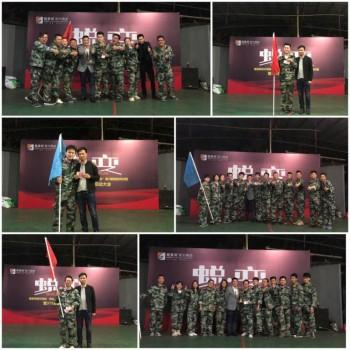烧烤晚宴在一片欢声笑语中落下帷幕！有聚也有散，格莱美的家人们，但下次相聚不会远的！

格莱美的家人们，准备好了吗？让我们一起在接下来的“315买砖不用钱”全国联动活动中打一场“漂亮”的战役，一“战”惊人吧！`声明：本文由入驻焦点开放平台的作者撰写，除焦点官方账号外，观点仅代表作者本人，不代表焦点立场错误信息举报电话： 400-099-0099，邮箱：jubao@vip.sohu.com，或点此进行意见反馈，或点此进行举报投诉。`A B C D E F G H J K L M N P Q R S T W X Y Z
A - B - C - D - E
• A
• 鞍山
• 安庆
• 安阳
• 安顺
• 安康
• 澳门
• B
• 北京
• 保定
• 包头
• 巴彦淖尔
• 本溪
• 蚌埠
• 亳州
• 滨州
• 北海
• 百色
• 巴中
• 毕节
• 保山
• 宝鸡
• 白银
• 巴州
• C
• 承德
• 沧州
• 长治
• 赤峰
• 朝阳
• 长春
• 常州
• 滁州
• 池州
• 长沙
• 常德
• 郴州
• 潮州
• 崇左
• 重庆
• 成都
• 楚雄
• 昌都
• 慈溪
• 常熟
• D
• 大同
• 大连
• 丹东
• 大庆
• 东营
• 德州
• 东莞
• 德阳
• 达州
• 大理
• 德宏
• 定西
• 儋州
• 东平
• E
• 鄂尔多斯
• 鄂州
• 恩施
F - G - H - I - J
• F
• 抚顺
• 阜新
• 阜阳
• 福州
• 抚州
• 佛山
• 防城港
• G
• 赣州
• 广州
• 桂林
• 贵港
• 广元
• 广安
• 贵阳
• 固原
• H
• 邯郸
• 衡水
• 呼和浩特
• 呼伦贝尔
• 葫芦岛
• 哈尔滨
• 黑河
• 淮安
• 杭州
• 湖州
• 合肥
• 淮南
• 淮北
• 黄山
• 菏泽
• 鹤壁
• 黄石
• 黄冈
• 衡阳
• 怀化
• 惠州
• 河源
• 贺州
• 河池
• 海口
• 红河
• 汉中
• 海东
• 怀来
• I
• J
• 晋中
• 锦州
• 吉林
• 鸡西
• 佳木斯
• 嘉兴
• 金华
• 景德镇
• 九江
• 吉安
• 济南
• 济宁
• 焦作
• 荆门
• 荆州
• 江门
• 揭阳
• 金昌
• 酒泉
• 嘉峪关
K - L - M - N - P
• K
• 开封
• 昆明
• 昆山
• L
• 廊坊
• 临汾
• 辽阳
• 连云港
• 丽水
• 六安
• 龙岩
• 莱芜
• 临沂
• 聊城
• 洛阳
• 漯河
• 娄底
• 柳州
• 来宾
• 泸州
• 乐山
• 六盘水
• 丽江
• 临沧
• 拉萨
• 林芝
• 兰州
• 陇南
• M
• 牡丹江
• 马鞍山
• 茂名
• 梅州
• 绵阳
• 眉山
• N
• 南京
• 南通
• 宁波
• 南平
• 宁德
• 南昌
• 南阳
• 南宁
• 内江
• 南充
• P
• 盘锦
• 莆田
• 平顶山
• 濮阳
• 攀枝花
• 普洱
• 平凉
Q - R - S - T - W
• Q
• 秦皇岛
• 齐齐哈尔
• 衢州
• 泉州
• 青岛
• 清远
• 钦州
• 黔南
• 曲靖
• 庆阳
• R
• 日照
• 日喀则
• S
• 石家庄
• 沈阳
• 双鸭山
• 绥化
• 上海
• 苏州
• 宿迁
• 绍兴
• 宿州
• 三明
• 上饶
• 三门峡
• 商丘
• 十堰
• 随州
• 邵阳
• 韶关
• 深圳
• 汕头
• 汕尾
• 三亚
• 三沙
• 遂宁
• 山南
• 商洛
• 石嘴山
• T
• 天津
• 唐山
• 太原
• 通辽
• 铁岭
• 泰州
• 台州
• 铜陵
• 泰安
• 铜仁
• 铜川
• 天水
• 天门
• W
• 乌海
• 乌兰察布
• 无锡
• 温州
• 芜湖
• 潍坊
• 威海
• 武汉
• 梧州
• 渭南
• 武威
• 吴忠
• 乌鲁木齐
X - Y - Z
• X
• 邢台
• 徐州
• 宣城
• 厦门
• 新乡
• 许昌
• 信阳
• 襄阳
• 孝感
• 咸宁
• 湘潭
• 湘西
• 西双版纳
• 西安
• 咸阳
• 西宁
• 仙桃
• 西昌
• Y
• 阳泉
• 运城
• 营口
• 盐城
• 扬州
• 鹰潭
• 宜春
• 烟台
• 宜昌
• 岳阳
• 益阳
• 永州
• 阳江
• 云浮
• 玉林
• 宜宾
• 雅安
• 玉溪
• 延安
• 榆林
• 银川
• Z
• 张家口
• 镇江
• 舟山
• 漳州
• 淄博
• 枣庄
• 郑州
• 周口
• 驻马店
• 株洲
• 张家界
• 珠海
• 湛江
• 肇庆
• 中山
• 自贡
• 资阳
• 遵义
• 昭通
• 张掖
• 中卫

1室1厅1厨1卫1阳台

1
2
3
4
5

0
1
2

1

1

0
1
2
3报名成功，资料已提交审核A B C D E F G H J K L M N P Q R S T W X Y Z
A - B - C - D - E
• A
• 鞍山
• 安庆
• 安阳
• 安顺
• 安康
• 澳门
• B
• 北京
• 保定
• 包头
• 巴彦淖尔
• 本溪
• 蚌埠
• 亳州
• 滨州
• 北海
• 百色
• 巴中
• 毕节
• 保山
• 宝鸡
• 白银
• 巴州
• C
• 承德
• 沧州
• 长治
• 赤峰
• 朝阳
• 长春
• 常州
• 滁州
• 池州
• 长沙
• 常德
• 郴州
• 潮州
• 崇左
• 重庆
• 成都
• 楚雄
• 昌都
• 慈溪
• 常熟
• D
• 大同
• 大连
• 丹东
• 大庆
• 东营
• 德州
• 东莞
• 德阳
• 达州
• 大理
• 德宏
• 定西
• 儋州
• 东平
• E
• 鄂尔多斯
• 鄂州
• 恩施
F - G - H - I - J
• F
• 抚顺
• 阜新
• 阜阳
• 福州
• 抚州
• 佛山
• 防城港
• G
• 赣州
• 广州
• 桂林
• 贵港
• 广元
• 广安
• 贵阳
• 固原
• H
• 邯郸
• 衡水
• 呼和浩特
• 呼伦贝尔
• 葫芦岛
• 哈尔滨
• 黑河
• 淮安
• 杭州
• 湖州
• 合肥
• 淮南
• 淮北
• 黄山
• 菏泽
• 鹤壁
• 黄石
• 黄冈
• 衡阳
• 怀化
• 惠州
• 河源
• 贺州
• 河池
• 海口
• 红河
• 汉中
• 海东
• 怀来
• I
• J
• 晋中
• 锦州
• 吉林
• 鸡西
• 佳木斯
• 嘉兴
• 金华
• 景德镇
• 九江
• 吉安
• 济南
• 济宁
• 焦作
• 荆门
• 荆州
• 江门
• 揭阳
• 金昌
• 酒泉
• 嘉峪关
K - L - M - N - P
• K
• 开封
• 昆明
• 昆山
• L
• 廊坊
• 临汾
• 辽阳
• 连云港
• 丽水
• 六安
• 龙岩
• 莱芜
• 临沂
• 聊城
• 洛阳
• 漯河
• 娄底
• 柳州
• 来宾
• 泸州
• 乐山
• 六盘水
• 丽江
• 临沧
• 拉萨
• 林芝
• 兰州
• 陇南
• M
• 牡丹江
• 马鞍山
• 茂名
• 梅州
• 绵阳
• 眉山
• N
• 南京
• 南通
• 宁波
• 南平
• 宁德
• 南昌
• 南阳
• 南宁
• 内江
• 南充
• P
• 盘锦
• 莆田
• 平顶山
• 濮阳
• 攀枝花
• 普洱
• 平凉
Q - R - S - T - W
• Q
• 秦皇岛
• 齐齐哈尔
• 衢州
• 泉州
• 青岛
• 清远
• 钦州
• 黔南
• 曲靖
• 庆阳
• R
• 日照
• 日喀则
• S
• 石家庄
• 沈阳
• 双鸭山
• 绥化
• 上海
• 苏州
• 宿迁
• 绍兴
• 宿州
• 三明
• 上饶
• 三门峡
• 商丘
• 十堰
• 随州
• 邵阳
• 韶关
• 深圳
• 汕头
• 汕尾
• 三亚
• 三沙
• 遂宁
• 山南
• 商洛
• 石嘴山
• T
• 天津
• 唐山
• 太原
• 通辽
• 铁岭
• 泰州
• 台州
• 铜陵
• 泰安
• 铜仁
• 铜川
• 天水
• 天门
• W
• 乌海
• 乌兰察布
• 无锡
• 温州
• 芜湖
• 潍坊
• 威海
• 武汉
• 梧州
• 渭南
• 武威
• 吴忠
• 乌鲁木齐
X - Y - Z
• X
• 邢台
• 徐州
• 宣城
• 厦门
• 新乡
• 许昌
• 信阳
• 襄阳
• 孝感
• 咸宁
• 湘潭
• 湘西
• 西双版纳
• 西安
• 咸阳
• 西宁
• 仙桃
• 西昌
• Y
• 阳泉
• 运城
• 营口
• 盐城
• 扬州
• 鹰潭
• 宜春
• 烟台
• 宜昌
• 岳阳
• 益阳
• 永州
• 阳江
• 云浮
• 玉林
• 宜宾
• 雅安
• 玉溪
• 延安
• 榆林
• 银川
• Z
• 张家口
• 镇江
• 舟山
• 漳州
• 淄博
• 枣庄
• 郑州
• 周口
• 驻马店
• 株洲
• 张家界
• 珠海
• 湛江
• 肇庆
• 中山
• 自贡
• 资阳
• 遵义
• 昭通
• 张掖
• 中卫• 手机• 分享
• 设计
免费设计
• 计算器
装修计算器
• 联系
• 置顶
返回顶部